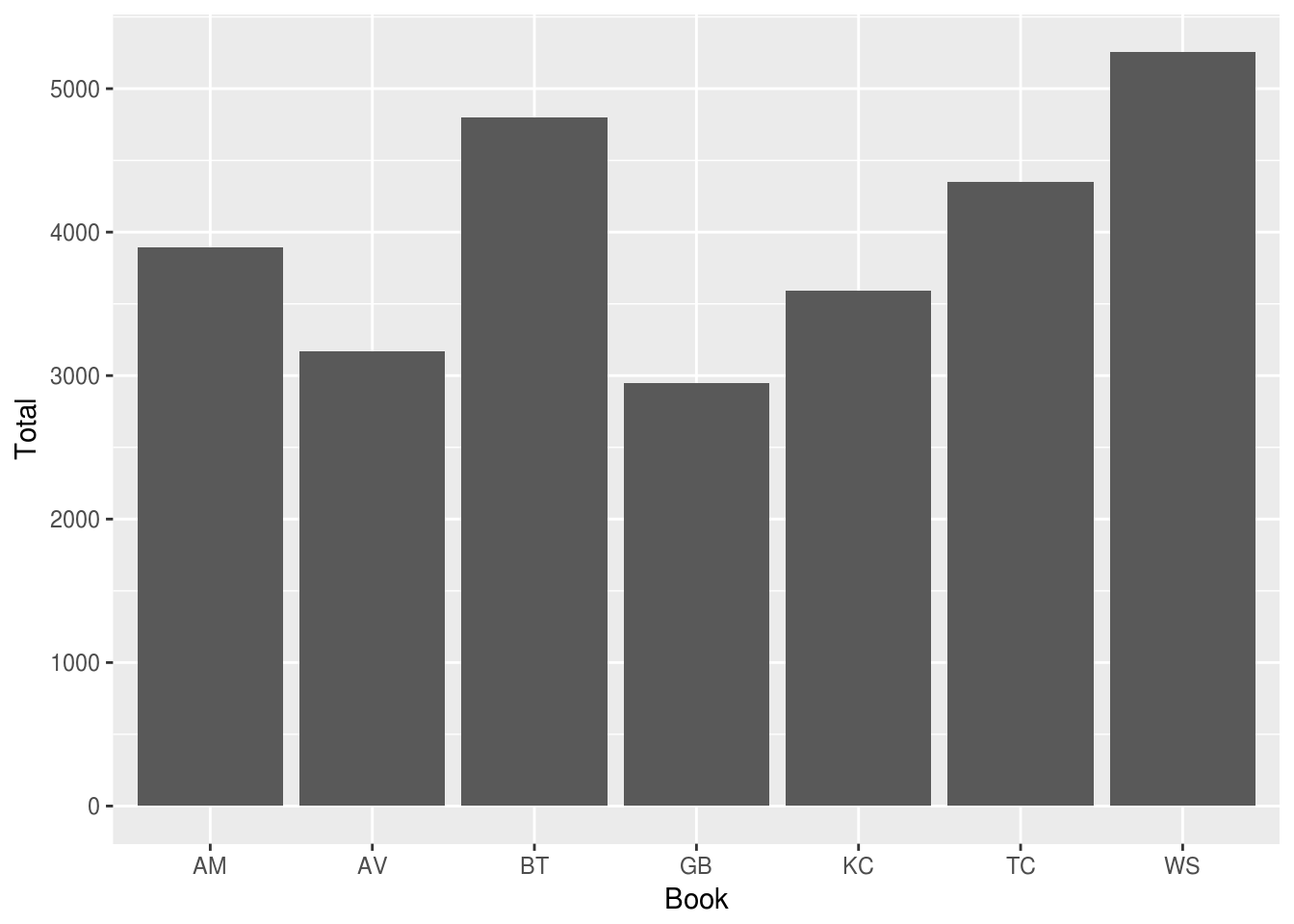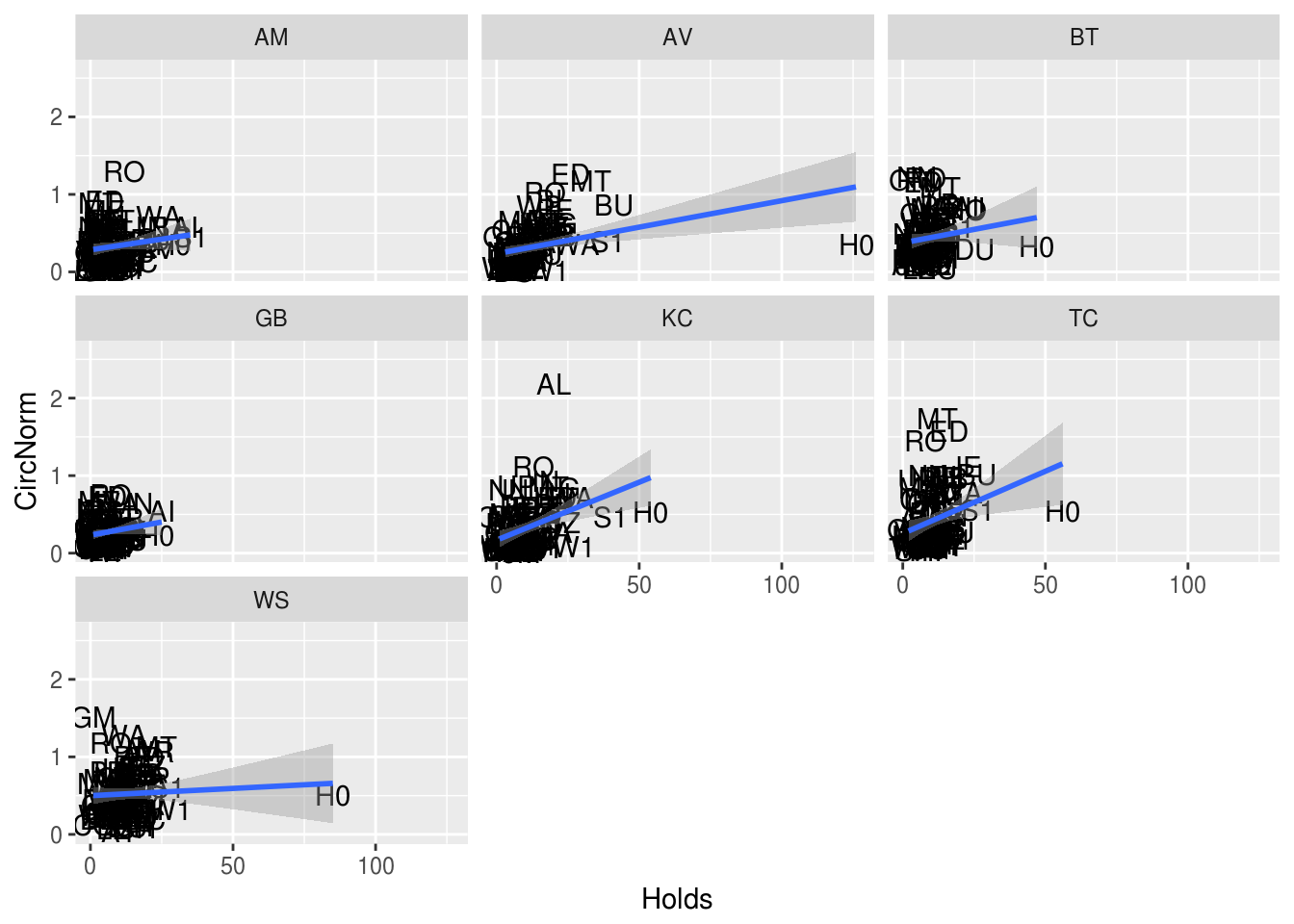This file computes a multi-level linear model of the circulation per 1k visitors data against the library data represented as the principal components of the demographic representation and the book holdings.

``````library(knitr)
setwd("/home/rburke/oboc/src/rcr-analysis/src/model/")

### Project constants

``````# Load project constants
setwd("/home/rburke/oboc/src/rcr-analysis/src")
source("common.R")``````

``````# Load libraries
library(plyr)
library(dplyr)``````
``````##
## Attaching package: 'dplyr'``````
``````## The following objects are masked from 'package:plyr':
##
##     arrange, count, desc, failwith, id, mutate, rename, summarise,
##     summarize``````
``````## The following objects are masked from 'package:stats':
##
##     filter, lag``````
``````## The following objects are masked from 'package:base':
##
##     intersect, setdiff, setequal, union``````
``````library(reshape2)
library(multilevel)``````
``## Loading required package: nlme``
``````##
## Attaching package: 'nlme'``````
``````## The following object is masked from 'package:dplyr':
##
##     collapse``````
``## Loading required package: MASS``
``````##
## Attaching package: 'MASS'``````
``````## The following object is masked from 'package:dplyr':
##
##     select``````
``library(caret)``
``## Loading required package: lattice``
``## Loading required package: ggplot2``
``library(lme4)``
``## Loading required package: Matrix``
``````##
## Attaching package: 'lme4'``````
``````## The following object is masked from 'package:nlme':
##
##     lmList``````
``````library(sjstats)
library(cvTools)``````
``## Loading required package: robustbase``
``library(merTools)``
``## Loading required package: arm``
``````##
## arm (Version 1.10-1, built: 2018-4-12)``````
``## Working directory is /home/rburke/oboc/src/rcr-analysis/src/model``
``library(RMySQL)``
``## Loading required package: DBI``
``library(ggplot2)``

### Library data including principal components

Note that the branch data does not include 2016 circulation

``````# Read the principal components of the demographic data
path.demo <- paste(RCR_DATA, "branch/branch-data-prcomp-",
DATA_VERSION, ".csv", sep="")
# Drop the parts that we don't use
demo <- demo[,c("Code", "CR_2011", "CR_2012", "CR_2013", "CR_2014", "CR_2015",
"PC1", "PC2", "PC3", "PC4", "PC5", "PC6")]

# Copy 2015 circulation into 2016. Need to update branch data.

demo\$CR_2016 <- demo\$CR_2015``````

### Connect to database

``````# Database connection
con <- dbConnect(MySQL(),
dbname="oboc", host="localhost")``````

### OBOC circulation data

``````# Circulation query
# Note that the normalized table has only the selected books and only the checkouts.
circ_query <- paste("select count(T.id_item), T.abbrev_season, T.code_branch ",
"from V_norm_trans2 T ",
"where day_season >= 0 and day_season < 365 ",
"group by T.abbrev_season, T.code_branch", sep="")
circ <- dbGetQuery(con, circ_query)
#circ <- dbFetch(rs1)
colnames(circ) <- c("Freq", "Book", "Code")``````

### Holdings data

``````# Read the total holdings data
holds_query <-
paste("select O.abbrev_season, R.code_branch, count(H.id_holding) ",
"from CPL_branch R, CPL_holding H, OBOC_season O, CPL_book B ",
"where H.book_holding = B.id_book and B.oboc_type_book = 'Y' and ",
"B.season_book = O.id_season and R.id_branch = H.branch_holding and ",
"(H.date_holding <= (O.launch_date_season + 365) or H.date_holding is null) ",
"group by O.abbrev_season, R.code_branch", sep="")
hold <- dbGetQuery(con, holds_query)
colnames(hold) <- c("Book", "Code", "Holds")``````

### Get book-year table from the database

``````year_query = "select O.abbrev_season, extract(year from O.launch_date_season) from OBOC_season O"
book.year <- dbGetQuery(con, year_query)
colnames(book.year) <- c("Book", "Year")``````

### Compute book-wise branch circulation

As it turns out, this series is pretty closely correlated with the library holdings for each book. (Perhaps not surprising as this is likely how titles were/are allocated.)

Scale to the range closer to the principal component values

``````# Extract total circulation data and associate by book-year
circ.year1 <- demo[,c("Code", "CR_2011", "CR_2012", "CR_2013",
"CR_2014", "CR_2015", "CR_2016")]
colnames(circ.year1) <- c("Code", "2011", "2012", "2013", "2014", "2015", "2016")
circ.year2 <- melt(circ.year1, id.vars="Code")
colnames(circ.year2) <- c("Code", "Year", "TotalCirc")
circ.year2\$CircS <- as.vector(scale(circ.year2\$TotalCirc, center=F, scale=T))
circ.year <- join(circ.year2, book.year, by="Year", type="left")
# The BA branch was closed in 2012
circ.year[is.na(circ.year\$CircS),]\$CircS <- 0
# Drop Year and TotalCirc columns
circ.year <- circ.year[,c("Code", "Book", "CircS")]``````

### Compute book-wise branch visitors

Scale to the range closer to the principal component values

``````# Read the visitor data
path.visit <- paste(RCR_DATA, "branch/visitor-count.csv", sep="")
# Create 2016 data by copying 2015 data
visit2016 <- visit[visit\$Year==2015,]
visit2016\$Year <- 2016
visitwith2016 <- rbind(visit, visit2016)
# This replaces NA values with the mean over the non-NA years
visit.noNA <- visitwith2016 %>% dplyr::group_by(Code) %>%
dplyr::mutate(YTD = replace(YTD, is.na(YTD), mean(YTD, na.rm=TRUE)))
visit.year <- join(as.data.frame(visit.noNA), book.year, by="Year", type="left")
# Drop Year column
visit.year <- visit.year[,c("Code", "Book", "YTD")]
visit.year\$VisitS <- as.vector(scale(visit.year\$YTD, center=F, scale=T))``````

### Perform joins

``````# Join book / branch event data for circulation, frequency and holdings
eventdf <- join(circ, hold, by=c("Code", "Book"))
eventdf <- join(eventdf, visit.year, by=c("Code", "Book"))
eventdf <- join(eventdf, circ.year, by=c("Code", "Book"))
fulldf <- join(eventdf, demo, by=c("Code"), type="left")``````

### Create data subsets

PW is dropped because it has no visitor data on the City of Chicago site. We create a square transform of PC2. This produces a more linear response in the circulation values.

``````# Drop PW, which has no visitor data for some reason
fulldf <- fulldf[!fulldf\$Code=="PW",]
# Compute circulation per 1k visitors
fulldf\$CircNorm <- fulldf\$Freq / fulldf\$YTD* 1000
# Transformed PC2 by squaring
fulldf\$PC2X <- fulldf\$PC2*fulldf\$PC2/5.0``````

Total circulation

``````bookcount <- fulldf %>% dplyr::group_by(Book) %>% dplyr::summarize(Total = sum(Freq))

p <- ggplot(bookcount, aes(x=Book, y=Total))
p <- p + geom_bar(stat="identity")
print(p)``````## Lattice plots full data

Plot the checkout frequency against the model variables.

### Holdings

``````# lattice plots of features
p <- ggplot(data=fulldf, aes(x=Holds, y=CircNorm, label=Code))
p <- p + geom_text() + geom_smooth(method="lm")
p <- p + facet_wrap(~Book)
print(p)``````
``## Warning: Removed 5 rows containing non-finite values (stat_smooth).``
``## Warning: Removed 5 rows containing missing values (geom_text).``### Total branch visitors

``````p <- ggplot(data=fulldf, aes(x=VisitS, y=CircNorm, label=Code))
p <- p + geom_text() + geom_smooth(method="lm")
p <- p + facet_wrap(~Book)
print(p)``````